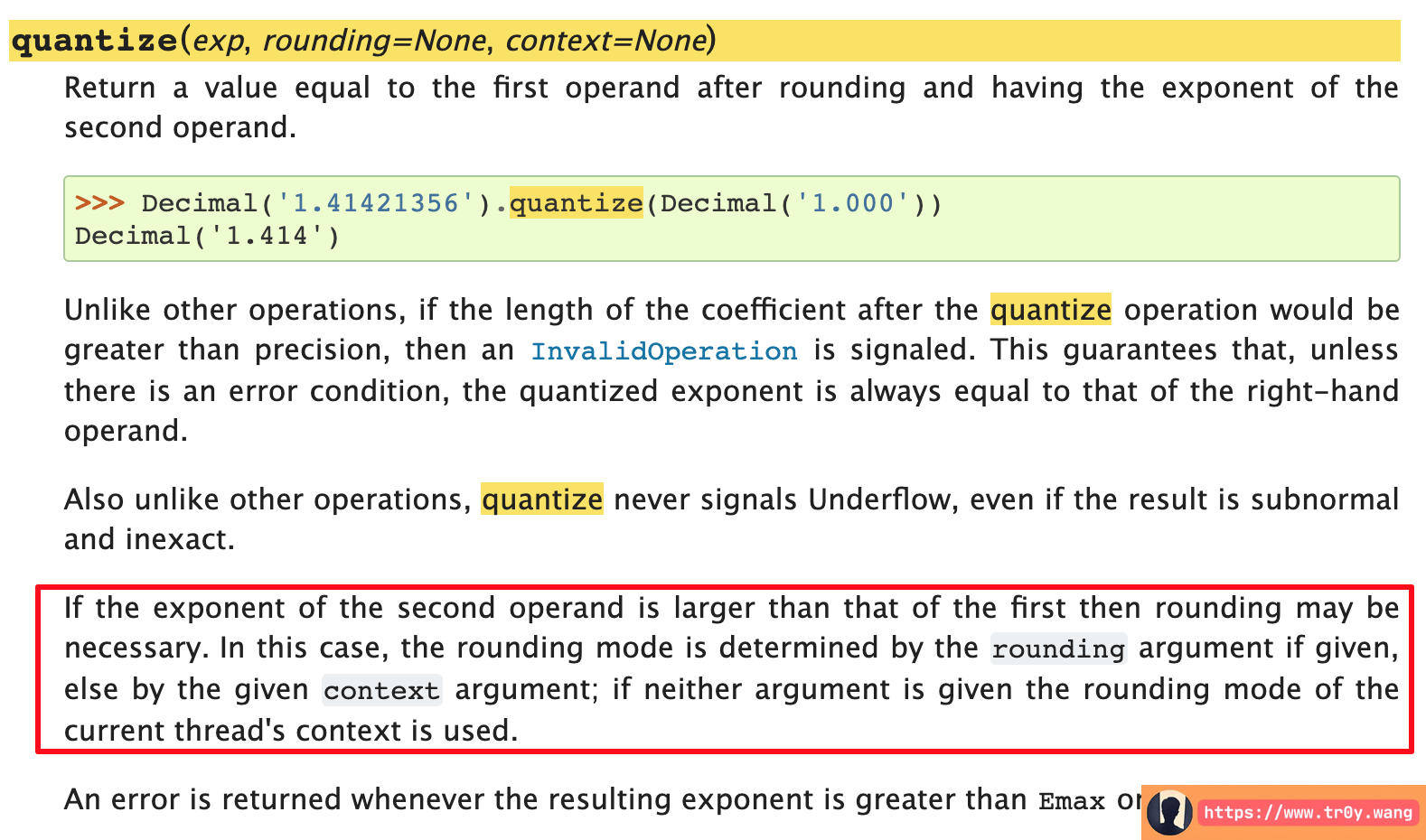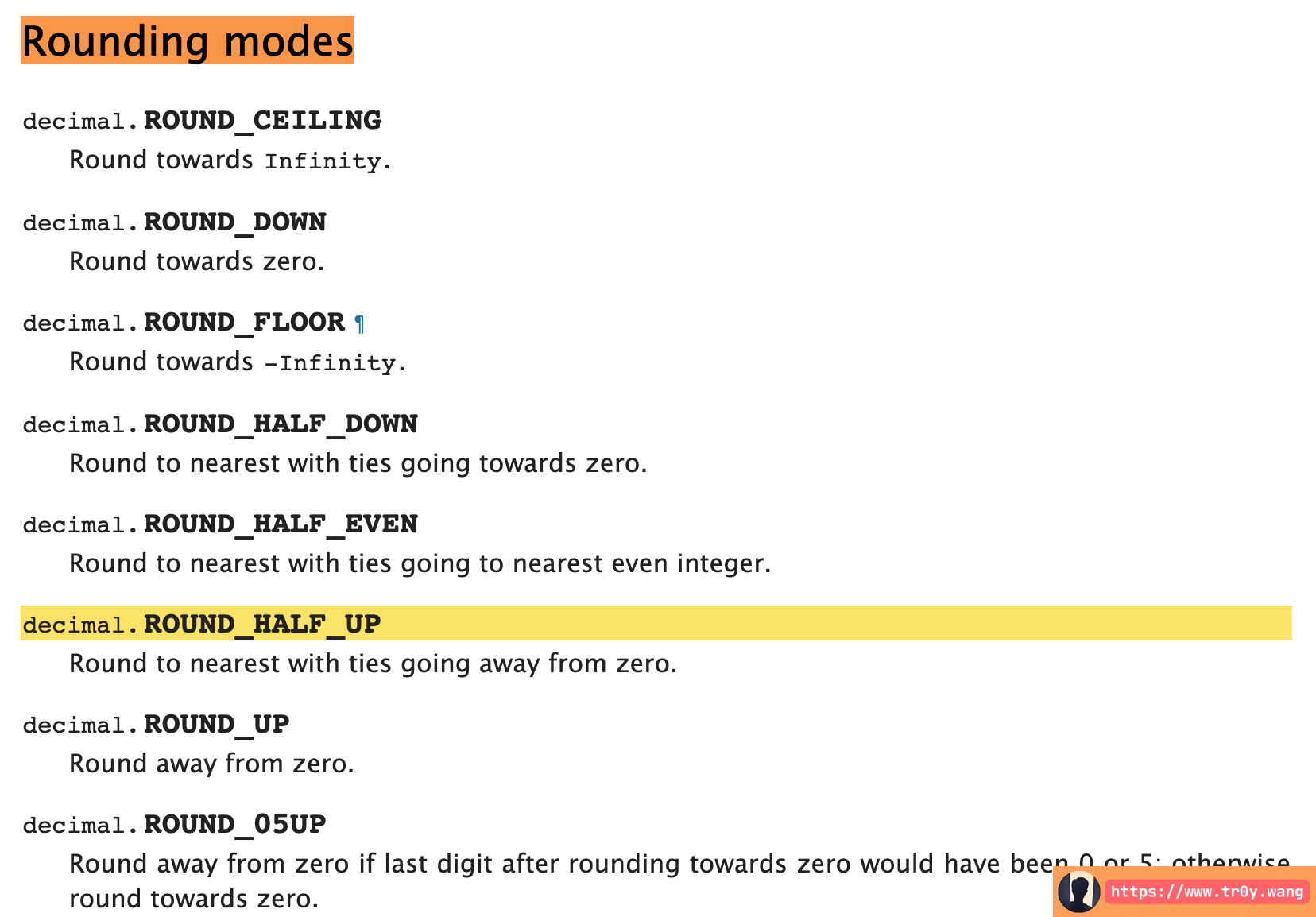Tr0y 4月 08, 2019 19:00:55 本文共 1.2k 字
• 文为知己者书
•• 在其它设备中阅读本文章
•round 函数的问题

In : round(1.115, 2)
Out: 1.11 # 错误，应该是 1.12

In : round(0.375, 2)
Out: 0.38 # 正确

In : from decimal import Decimal # decimal 是 Python 专门处理高精度的库

In : Decimal(1.115)
Out: Decimal('1.1149999999999999911182158029987476766109466552734375')

In : Decimal(1.125)
Out: Decimal('1.125')

In : round(1.125, 2)
Out: 1.12

1. 如果 d<5，直接舍去
2. 如果 d<5，直接进位
3. 如果 d == 5：
1. d 后面还有非 0 数字，例如 a.bcdef，f 非 0，那么要进位
2. d 后面没有数据，且 c 为偶数，那么不进位
3. d 后面没有数据，且 c 为奇数，那么要进位

1. 这个数可能无法被计算机精确地表示，这个误差是否能接受？
2. 奇进偶舍这个方法的误差是否能接受？

decimal: 高精度解决方案

In : Decimal(1.115)
Out: Decimal('1.1149999999999999911182158029987476766109466552734375')

In : Decimal('1.115')
Out: Decimal('1.115')

In : Decimal('1.115').quantize(Decimal('0.00'))
Out: Decimal('1.12')

In : Decimal('0.125').quantize(Decimal('0.00'))
Out: Decimal('0.12')

quantizeIn : from decimal import getcontext

In : getcontext().rounding
Out: 'ROUND_HALF_EVEN'

ROUND_HALF_EVENRound to nearest with ties going to nearest even integer.，就是我们上面提到的 奇进偶舍

ROUND_HALF_UP 才是我们想要的：

In : import decimal

In : Decimal('1.115').quantize(Decimal('0.00'), rounding=decimal.ROUND_HALF_UP)
Out: Decimal('1.12')

In : Decimal('0.125').quantize(Decimal('0.00'), rounding=decimal.ROUND_HALF_UP)
Out: Decimal('0.13')

int(0.115*100+0.5)/100

2 为就是 100，3 位就是 1000，以此类推。我拿到 Python 里试了一下，还真的可以：

In : int(0.115*100+0.5)/100
Out: 0.12

In : int(0.125*100+0.5)/100
Out: 0.13

End

What do you think?